# NCERT Solutions for Class 6 Maths Chapter 7 Fractions

Here, we are providing Chapter 7 Fractions NCERT Solutions for Class 6 Maths which is very helpful in understanding the concepts in practical way. You can also complete your homework on time through the help of these Chapter 7 NCERT Solutions and able to solve the difficult problems in a given in a exercise. Also, you can download PDF of NCERT Solutions for Class 9 Maths Chapter 7 Fractions that will help in achieving more marks.

Fractions NCERT Solutions will help an individual to increase concentration and you can solve questions of supplementary books easily. It is very essential for scoring good marks in the class test or board examinations.Exercise 7.1

1. Write the fraction representing the shaded portion:(i)2/4
(ii) 8/9
(iii) 4/8
(iv)1/4
(v)3/7
(vi)9/12
(vii)10/10
(viii)4/9
(ix)4/8

(x)1/2

2. Colour the part according to the given fraction: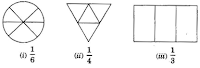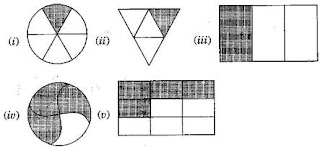3. Identify the error, if any?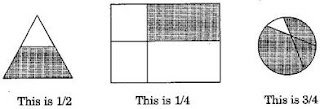All the figures are not equally divided. For making fractions, it is necessary that figure is divided into equal parts.

4. What fraction of a day is 8 hours?

Since, 1 day = 24 hours.
Therefore, the fraction of 8 hours = 8/24=1/3

5. What fraction of an hour is 40 minutes?

Since, 1 hour = 60 minutes.
Therefore, the fraction of 40 minutes = 40/60=2/3

6. Arya, Abhimanyu and Vivek shared lunch. Arya has brought two sandwiches, one made of vegetable and one of jam. The other two boys forgot to bring their lunch. Arya agreed to share his sandwiches so that each person will have an equal share of each sandwich.
(a)How can Arya divide his sandwiches so that each person has an equal share?
(b)What part of a sandwich will each boy receive?

(a) Arya will divide each sandwich into three equal parts and give one part of each sandwich to each one of them.
(b) 1×1/3=1/3

7. Kanchan dyes dresses. She had to dye 30 dresses. She has so far finished 20 dresses. What fraction of dresses has she finished?

Total number of dresses to dye= 30
Work completed = 20

Fraction of completed work = 20/30=2/3

8. Write the natural numbers from 2 to 12. What fraction of them are prime numbers?

Natural numbers from 2 to 12: 2, 3, 4, 5, 6, 7, 8, 9, 10, 11, 12
Prime numbers from 2 to 12: 2, 3, 5, 7, 11

Hence, fraction of prime numbers = 5/11

9. Write the natural numbers from 102 to 113. What fraction of them are prime numbers?

Natural numbers from 102 to 113: 102, 103, 104, 105, 106, 107, 108, 109, 110, 111, 112, 113
Prime numbers from 102 to 113: 103, 107, 109, 113

Hence fraction of prime numbers = 4/12=1/3

10. What fraction of these circles has ‘X’s in them?Total number of circles = 8 and number of circles having ‘X’ = 4
Hence, the fraction = 4/8

11. Kristin received a CD player for her birthday. She bought 3 CDs and received 5 others as gifts. What fraction of her total CDs did she buy and what fraction did she receive as gifts?

Total number of CDs = 3 + 5 = 8
Number of CDs purchased = 3
Fraction of CDs purchased = 3/8

Exercise 7.2

1. Draw number lines and locate the points on them:
(a)1/2,1/4,3/4,4/4
(b)1/8,2/8,3/8,7/8
(c)2/5,3/5,8/5,4/5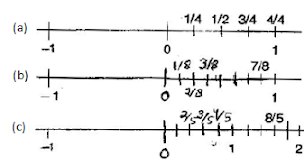2. Express the following fractions as mixed fractions:
(a)20/3
(b)11/5
(c)17/7
(d)28/5
(e)19/6
(f)35/9

(a)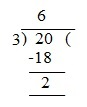20/3= 6.2/3

(b)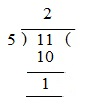∴11/5= 2.1/5

(c)∴17/7= 2.3/7

(d)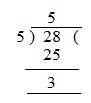∴28/5=5.3/5

(e)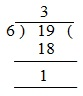19/6=3.1/6

(f)∴35/9=3.8/9

3. Express the following as improper fractions:
(a)7.3/4
(b)5.6/7
(c)2.5/6
(d)10.3/5
(e)9.3/7
(f)8.4/9

(a) 7.3/4 = {(7×4)+3}/4 = (28+3)/4 = 31/4
(b) 5.6/7 = {(5×7)+6}/7 = (35+6)/7 = 41/7
(c) 2.5/6 = {(2×6)+5}/6 = (12+5)/6 =17/6
(d) 10.3/5 = {(10×5)+3}/5 = (50+3)/5 = 53/5
(e) 9.3/7 = {(9×7)+3}/7= (63+3)/7 = 66/7
(f) 8.4/9 = {(8×9)+4}/9 = (72+4)/9 = 76/9

Exercise 7.3

1. Write fractions. Are all these fractions equivalent:
(a)(b)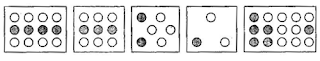(a) 1/2,2/4,3/6,4/8

Yes, all of these fractions are equivalent.

(b) 4/12,3/9,2/6,1/3,6/15
No, these fractions are not equivalent.

2. Write the fraction and pair up the equivalent fractions to each row:

(a) 1/2
(ii) 4/8=1/2

(b) 4/6=2/3
(iv) 8/12=2/3

(c) 3/9=1/3
(i) 6/18=1/3

(d) 2/8=1/4
(v) 4/16=1/4

(e) 1/4

(iii) 12/16

3. Replace __ in each of the following by the correct number:
(a) 2/7=8/__
(b) 5/8=10/__
(c) 3/5= __/20
(d) 45/60=15/__
(e) 18/24=__/ 4

(a) 2/7 = 2×4/7×4 = 8/28
(b) 5/8 = 5×2/8×2 = 10/16
(c) 3/5 = 3×4/5×4 = 12/20
(d) 45/60 = 45÷3/60÷3=15/20

(e) 18/24 = 18÷6/24÷6 = 3/4

4. Find the equivalent fraction of 3/5 having:
(a) denominator 20
(b) numerator 9
(c) denominator 30
(d) numerator 27

(a) 3/5 =3×4/5×4=12/20
(b) 3/5=3×3/5×3=9/15
(c) 3/5=3×6/5×6=18/30

(d) 3/5=3×9/5×9=27/45

5. Find the equivalent fraction of 36/48 with:
(a) numerator 9
(b) denominator 4

(a) 36/48 = 36÷4/48÷4 = 9/12
(b) 36/48 = 36÷12/48÷12 = 3/4

6. Check whether the given fraction are equivalent:
(a) 5/9,30/54
(b) 3/10,12/50
(c)7/13,5/11

(a) 5/9,30/54 = 5×6/9×6,30/54= 30/54, 30/54
Therefore, 5/9,30/54 are equivalent.

(b) 3/10,12/50= 3×5/10×5,12/50= 15/50,12/50
Therefore, 3/10,12/50 are not equivalent.

(c) 7/13,5/11 = 7×11/13×11,5×13/11×13 = 77/143,65/143

Therefore, 7/13,5/11 are not equivalent fraction.

7. Reduce the following fractions to simplest form:
(a) 48/60
(b) 150/60
(c) 84/98
(d) 12/52
(e) 7/28

(a) 48/60= (2×2×2×2×3)/(2×2×3×5) =4/5
(b) 150/60= (3×5×10)/(2×3×10)= 5/2
(c) 84/98= (2×3×14)/(7×14) = 6/7
(d) 12/52= (2×2×3)/(2×2×13) =3/13

(e) 7/28=7/(2×2×7)= 1/4

8. Ramesh had 20 pencils, Sheelu had 50 pencils and Jamaal had 80 pencils. After 4 months, Ramesh used up 10 pencils, Sheelu used up 25 pencils and Jamaal used up 40 pencils. What fraction did each use up? Check whether each has used an equal fraction of her/his pencils?

Ramesh: Total pencils = 20
Pencils used = 10
Fraction = 10/20 =1/2
Sheelu: Total pencils = 50
Pencils used = 25
Fraction = 25/50=1/2
Jamaal: Total pencils = 80
Pencils used = 40
Fraction = 40/80= 1/2

Since, all of them used half of their pencils, therefore each one of them used equal fraction of pencils.

9. Match the equivalent fractions and write two more for each:
 (i)250/400 (a) 2/3 (ii)180/200 (b) 2/5 (iii)660/990 (c) 1/2 (iv)180/360 (d) 5/8 (v)220/550 (e) 9/10

(i) 250/400=5/8,10/16,15/24
(d) 5/8

(ii) 180/200=9/10,18/20,27/30
(e) 9/10

(iii) 660/990=2/3,4/6,6/96
(a) 2/3

(iv) 180/360=1/2,2/4,3/6
(c)1/2

(v) 220/550 = 2/5, 4/10, 6/15
(b) 2/5

Exercise 7.4

1. Write shaded portion as fraction. Arrange them in ascending and descending order using correct sign ‘<’, ‘>’, ‘=’ between the fractions:
(a)(b)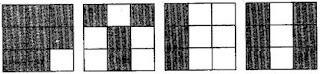(c) Show 2/6,4/6,8/6 and 6/6on the number line. Put appropriate signs between the fractions given:
5/6 - 2/6, 3/6 - 0
1/6 - 6/6, 8/6 - 5/6

(a) 3/8,6/8,4/8,1/8
Ascending order: 1/8<3/8<4/8<6/8
Descending order: 6/8>4/8>3/8>1/8

(b) 8/9,4/9,3/9,6/9
Ascending order: 3/9<4/9<6/9<8/9
Descending order: 8/9>6/9>4/9>3/9

(c) Number line5/6>2/6 1/6<6/6
3/6>0/6 8/6>5/6

2. Compare the fractions and put an appropriate sign:
(a) 3/6 - 5/6
(b) 1/7 - 1/4
(c) 4/5 - 5/5
(d) 3/5 - 3/7

(a) 3/6<5/6
(b) 1/7<1/4
(c) 4/5<5/5
(d) 3/5>3/7

3. Make five more pairs and put appropriate signs.

(a) 9/10>6/10
(b) 1/3>1/6
(c) 1/8<1/5
(d) 7/8<11/8
(e) 11/13>9/13

4. Look at the figures and write ‘<’ or ‘>’ between the given pairs of fractions:(a) 1/6 _1/3
(b) 3/4 _ 2/6
(c) 2/3 _ 2/4
(d) 6/6 _ 3/3
(e) 5/6 _5/5
Make five more such problems and solve them with your friends.
Five more such problems:
(a) 1/2 _ 3/6
(b) 2/3 _ 3/5
(c) 3/4 _ 4/6
(d) 5/6 _ 2/2
(e) 0/1 _ 0/6

(a) 1/6<1/3
(b) 3/4>2/6
(c) 2/3>2/4
(d) 6/6=3/3
(e) 5/6<5/5

Five more such problems solution:
(a) 1/2 = 3/6
(b) 2/3 > 3/5
(c) 3/4 > 4/6
(d) 5/6 < 2/2
(e) 0/1 = 0/6

5. How quickly can you do this? Fill the appropriate sign (<, =, >):
(a) 1/2 _ 1/5
(b) 2/4 _3/6
(c) 3/5 _ 2/3
(d) 3/4 _ 2/8
(e) 3/5 _ 6/5
(f) 7/9 _ 3/9
(g) 1/4 _ 2/8
(h) 6/10_ 4/5
(i) 3/4 _ 7/8
(j) 6/10 _ 4/5
(k) 5/7 _ 15/21

(a) 1/2 > 1/5
(b) 2/4 = 3/6
(c) 3/5 < 2/3
(d) 3/4 > 2/8
(e) 3/5 < 6/5
(f) 7/9 > 3/9
(g) 1/4 = 2/8
(h) 6/10 < 4/5
(i) 3/4 < 7/8
(j) 6/10 < 4/5
(k) 5/7 = 15/21

6. The following fractions represent just three different numbers. Separate them into three groups of equivalent fractions, by changing each one to its simplest form:
(a) 2/12
(b) 3/15
(c) 8/50
(d) 16/100
(e) 10/60
(f) 15/75
(g) 12/60
(h) 16/96
(i) 12/75
(j) 12/72
(k) 3/18
(l) 4/25

(a) 2/12=1/6
(b) 3/15=1/5
(c) 8/50=4/25
(d) 16/100=4/25
(e) 10/60=1/6
(f) 15/75=1/5
(g) 12/60=1/5
(h) 16/96=1/6
(i) 12/75=4/25
(j) 12/72=1/6
(k) 3/18=1/6
(l) 4/25=4/25
Equivalent groups:
I group: 1/5 [(b), (f), (g)]
II group: 1/6 [(a), (e), (h), (j), (k)]
III group: 4/25 [(c), (d), (i), (l)]

7. Find answers to the following. Write and indicate how you solved them:
(a) Is 5/9 equal to 4/5 ?
(b) Is 9/16equal to 5/9 ?
(c) Is 4/5 equal to 16/20 ?
(d) Is 1/15 equal to 4/30 ?

(a)5/9 and 4/5
⇒ 5×5/9×5 =25/45 and 4×9/5×9=36/45 [∵ L.C.M. of 9 and 5 is 45]
Since, 25/45≠36/45
Therefore, 5/9≠4/5

(b) 9/16 and 5/9
⇒ 9×9/16×9= 81/144 and 5×16/9×16=80/144 [∵ L.C.M. of 16 and 9 is 144]
Since, 81/144≠80/144
Therefore, 9/16≠5/9

(c) 4/5 and 16/20
⇒ 4×20/ 5×20=80/100 and 16×5/20×5=80/100 [∵ L.C.M. of 5 and 20 is 100]
Since, 80/100=80/100
Therefore, 4/5=16/20

(d) 1/15 and 4/30
⇒ 1×21/5×2=2/30 and 4×1/30×1=4/30 [∵ L.C.M. of 15 and 30 is 30]
Since, 2/30≠4/30
Therefore,

1/15≠4/30

8. Ila read 25 pages of a book containing 100 pages. Lalita read 2/5 of the same book. Who read less?

Ila read 25 pages out of 100 pages.
Fraction of reading the pages = 25/100=1/4th part of book
Lalita read 2/5th part of book = 40/100 pages
Since 1/4<2/5

9. Rafiq exercised for 3/6 of an hour, while Rohit exercised for 3/4 of an hour. Who exercised for a longer time?

Rafiq exercised 3/6 of an hour.
Rohit exercised 3/4 of an hour.
Since 3/4>3/6
Therefore, Rohit exercised for a longer time.

10. In a class A of 25 students, 20 passed in first class; in another class B of 30 students, 24 passed in first class. In which class was a greater fraction of students getting first class?

In class A, 20 passed out of 25, i.e., 20/25= 4/5
In class B, 24 passed out of 30, i.e., 24/30 = 4/5

Hence, each class have same fraction of student getting first class.

Exercise 7.5

1. Write the fractions appropriately as additions or subtractions(a) 1/5+2/5=(1+2)/5= 3/5
(b) 5/5−3/5=(5−3)/5=2/5
(c) 2/6+3/6=(2+3)/6=5/6

2. Solve:
(a) 1/18+1/18
(b) 8/15+3/15
(c) 7/7−5/7
(d) 1/22+21/22
(e) 12/15−7/15
(f) 5/8+3/8
(g) 1−2/3(1=3/3)
(h) 1/4+0/4
(i) 3−12/5

(a) 1/18+1/18=(1+1)/18=2/18=1/9
(b) 8/15+3/15=(8+3)/15=11/15
(c) 7/7−5/7=(7−5)/7=2/7
(d) 1/22+21/22=(1+21)/22=22/22=1
(e) 12/15−7/15=(12−7)/15=5/15=1/3
(f) 5/8+3/8=8/8=1
(g) 1−2/3=3/3−2/3=(3−2)/2=1/3
(h) 1/4+0/4=(1+0)/4=1/4
(i) 3−12/5=15/5−12/5=(15−12)/5=3/5

3. Shubham painted 2/3 of the wall space in his room. His sister Madhavi helped and painted 1/3 of the wall space. How much did they paint together?

Fraction of the wall painted by Shubham = 2/3
Fraction of the wall painted by Madhavi = 1/3
Total painting done by both of them = 2/3+1/3=(2+1)/3= 3/3=1
Therefore, they painted the wall completely.

4. Fill in the missing fractions:
(a) 7/10− __ = 3/10
(b) __ −3/21 =5/21
(c) __ −3/6=3/6
(d) __ +5/27=12/27

(a) 4/10
(b) 8/21
(c) 6/6
(d) 7/27

5. Javed was given a basket of 7 oranges, He sold only 5 oranges. What fraction of oranges was left in the basket?

Total = 1
Fraction of Orange left = 1−5/7
= 7/7−5/7=7−5/7=2/7
Thus, 2/7 oranges was left in the basket.

Exercise 7.6

1. Solve:
(a) 2/3+1/7
(b) 3/10+7/15
(c) 4/9+2/7
(d) 5/7+1/3
(e) 2/5+1/6
(f) 4/5+2/3
(g) 3/4−1/3
(h) 5/6−1/3
(i) 2/3+3/4+1/2
(j) 1/2+1/3+1/6
(k) 1.1/3+3.2/3
(l) 4.2/3+3.1/4
(m) 16/5−7/5
(n) 4/3−1/2

(a) L.C.M. of 3 and 7 is 21
∴ 2/3+1/7=(2×7+1×3)/21=(14+3)/21=17/21

(b) L.C.M. of 10 and 15 is 30
∴ 3/10+7/15=(3×3+7×2)/30=(9+14)/30=23/30

(c) L.C.M. of 9 and 7 is 63
∴ 4/9+2/7=(4×7+2×9)/63=(28+18)/63=46/63

(d) L.C.M. of 7 and 3 is 21
∴ 5/7+1/3=(5×3+7×1)/21=(15+7)/21=22/21=1.1/21

(e) L.C.M. of 5 and 6 is 30
∴ 2/5+1/6=(2×6+5×1)/30=(12+5)/30=17/30

(f) L.C.M. of 5 and 3 is 15
∴ 4/5+2/345+23 = (4×3+2×5)/15=(12+10)/15=22/15=1.7/15

(g) L.C.M. of 4 and 3 is 12
∴ 3/4−1/3=(3×3−4×1)/12=(9−4)/12=5/12

(h) L.C.M. of 6 and 3 is 6
∴ 5/6−1/3=(5×1−2×1)/6=(5−2)/6=3/6=1/2

(i) L.C.M. of 3, 4 and 2 is 12
∴ 2/3+3/4+1/2=(2×4+3×3+1×6)/12=(6+9+6)/12=23/12=1.11/12

(j) L.C.M. of 2, 3, and 6 is 6
∴ 1/2+1/3+1/6=(1×3+1×2+1×1)/6= (3+2+1)/6=6/6=1

(k) L.C.M. of 3 and 3 is 3
∴ 4/3+11/3=(4+11)/3=15/3=5

(l) L.C.M. of 3 and 4 is 12
∴ 14/3+13/4=(14×4+13×3)/12=(56+39)/12=95/12=7.11/12

(m) L.C.M. of 5 and 5 is 5
∴ 16/5−7/5=(16−7)/5=9/5=1.4/5

(n) L.C.M. of 3 and 2 is 6

∴ 4/3−1/2=(4×2−1×3)/6= (8−3)/6=5/6

2. Sarika bought 2/5 meter of ribbon and Lalita 3/4 meter of ribbon. What is the total length of the ribbon they bought?

Ribbon bought by Sarita = 2/5 m and Ribbon bought by Lalita = 3/4 m
Total length of the ribbon = 2/5+3/4= (2×4+5×3)/20 [∵ L.C.M. of 5 and 4 is 20]
= (8+15)/20=23/20=1.3/20 m
Therefore, they bought 1.3/20 m of ribbon.

3. Naina was given 1.1/2piece of cake and Najma was given 1.1/3piece of cake. Find the total amount of cake given to both of them.

Cake taken by Naina = 1.1/2 piece and Cake taken by Najma = 1.1/3 piece
Total cake taken = 1.1/2+ 1.1/3= 3/2+4/3 = (3×3+4×2) [∵ L.C.M. of 2 and 3 is 6]
= (9+8)/6=17/6=25/6
Therefore total consumption of cake is 25/6.

4. Fill in the boxes:
(a) ___ −5/8=1/4
(b)__ −1/5=1/2
(c) 1/2− __=1/6

(a) 1/4+5/8=(2+5)/8=7/8
(b) 1/2+1/5=(5+2)/10=7/10
(c) 1/2−1/6=(3−1)/6=2/6

5. Complete the addition – subtraction box: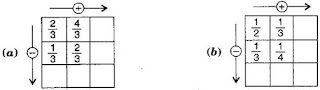6. A piece of wire 7/8meter long broke into two pieces. One piece was 1/4 meter long. How long is the other piece?

Total length of wire = 7/8 meter
Length of first part = 1/4 meter
Remaining part = 7/8−1/4=(7×1−2×1)/8 [∵ L.C.M. of 8 and 4 is 8]
= (7−2)/8=5/8meter

Therefore, the length of the remaining part is 5/8 meter.

7. Nandini house is 9/10 km from her school. She walked some distance and then took a bus for 1/2 km to reach the school. How far did she walk?

Total distance between the school and house = 9/10 km
Distance covered by bus = 1/2 km
Remaining distance = 9/10−1/2=(9×1−1×5)/10 [∵ L.C.M. of 10 and 2 is 10]
= (9−5)/10=4/10=2/5 km
Therefore, distance covered by walking us 2/5 km.

8. Asha and Samuel have bookshelves of the same size partly filled with books. Asha’s shelf is 5/6th full and Samuel’s shelf is 2/5th full. Whose bookshelf is more filled and by what fraction?

5/6 and 2/5
⇒ 5/6×5/5=25/30 and 2/5×6/6=12/30 [∵ L.C.M. of 6 and 5 is 30]
∵ 25/30>12/30 ⇒ 5/6>2/5
∴ Asha’s bookshelf is more covered than Samuel.
Difference = 25/30−12/30=13/30

9. Jaidev takes 2.1/5 minutes to walk across the school ground. Rahul takes 7/4 minutes to do the same. Who takes less time and by what fraction?

Time taken by Jaidev = 2.1/5 minutes = 11/5minutes
Time taken by Rahul = 7/4 minutes
Difference = 11/5−7/4= (11×4−7×5)/20 [∵ L.C.M. of 5 and 4 is 20]
= (44−35)/20=9/20 minutes
Thus, Rahul takes less time, which is 9/20 minutes.

## NCERT Solutions for Class 6 Maths Chapter 7 Fractions

Class 6 Maths NCERT Solutions are prerequisites before solving exemplar problems and going for supplementary Maths Books. A fraction is a part of a whole. A whole can be a group of objects or a single object. For example, 9/18 is a fraction. In this, 9 is called the numerator and 18 is called the denominator.

• A proper fraction is a number representing a part of a whole. In a proper fraction, the number in the denominator shows the number of parts into which the whole is divided, while the number in the numerator shows the number of parts that have been taken.

• A fraction in which the numerator is bigger than the denominator is called an improper fraction.

If you want exercisewise NCERT Solutions for Chapter 7 then you can get them below just by clicking on the links provided. It will make you understand the topics in most simple manner and grasp it easily to perform better.

• Exercise 7.1 Chapter 7 Class 6 Maths NCERT Solutions
• Exercise 7.2 Chapter 7 Class 6 Maths NCERT Solutions
• Exercise 7.3 Chapter 7 Class 6 Maths NCERT Solutions
• Exercise 7.4 Chapter 7 Class 6 Maths NCERT Solutions
• Exercise 7.5 Chapter 7 Class 6 Maths NCERT Solutions
• Exercise 7.6 Chapter 7 Class 6 Maths NCERT Solutions

These NCERT Solutions can help you in figuring out the latest marking scheme and prepare your answers. It help the students in learning complex topics and problems in an easy way. These will help you in improving the marks in the examinations and have edge over your classmates.

### NCERT Solutions for Class 6 Maths Chapters:

 Chapter 1 Knowing Our Numbers Chapter 2 Whole Numbers Chapter 3 Playing with Numbers Chapter 4 Basic Geometrical Ideas Chapter 5 Understanding Elementary Shapes Chapter 6 Integers Chapter 8 Decimals Chapter 9 Data Handling Chapter 10 Mensuration Chapter 11 Algebra Chapter 12 Ratio and Proportion Chapter 13 Symmetry Chapter 14 Practical Geometry

FAQ on Chapter 7 Fractions

#### How many exercises in Chapter 7 Fractions?

There are total 6 exercises in the chapter which allow students to cover the entire syllabus effectively without any frustration. It will make you well versed in variety of topics and you will be able to instantly recollect your ideas and shape your answers.

#### How to convert improper fraction into mixed fraction?

An improper fraction can be expressed as mixed fraction by dividing the numerator by the denominator of the improper fraction to obtain the quotient and the remainder.

#### What do you mean by simplest form of fraction?

A fraction is said to be in its simplest form or its lowest form if its numerator and denominator have no common factor except one. The simplest form of a given fraction can also be found by dividing its numerator and denominator by its highest common factor (HCF).

#### What is Unlike Fractions and how to add them?

Fractions with different numbers in the denominators are said to be unlike fractions.